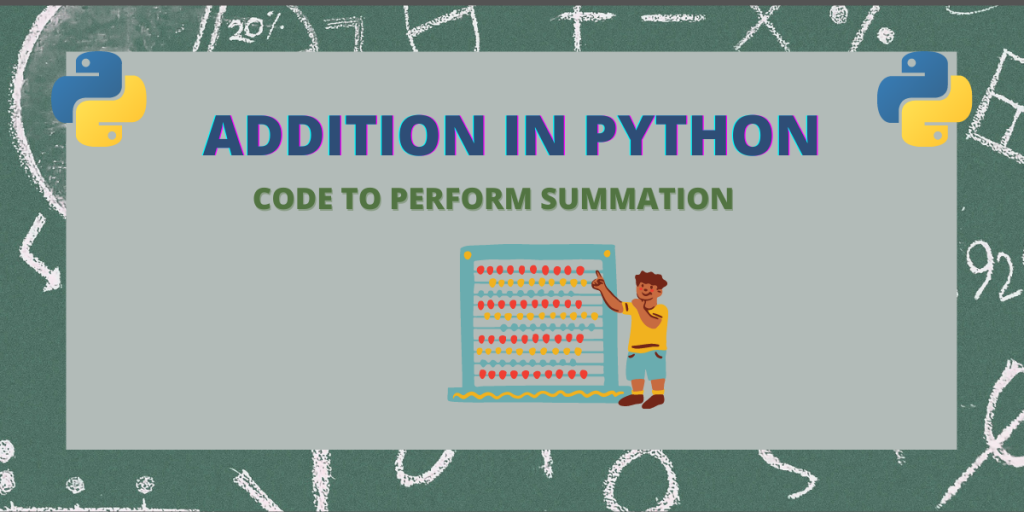# How to Perform Addition in Python?In this article, we’ll go over one of the most basic topics. This is good for you if you are a beginner. But if you have already coded in Python, skip this.

Also read: The sum() method in Python

## Addition in Python with two numbers from user input

We’ll be using the input() method to accept user input and then use those numbers to perform addition in Python. The basic code for addition of two numbers in python is:

```def adding(x , y):
return x + y

a = int(input("Enter first number :" ))
b = int(input("Enter second number :"))

print("addition of {} and {} is {}".format(a,b,sum))
```

The output is :

```Enter first number : 6
Enter second number : 5
Addition of 6 and 5 is 11
```

In the above code, we’ve typecasted the input to int using the int() method to convert the string input to integer for our processing.

## Perform Addition Operations on Elements of a List

We can add all the items of a list using loop as shown below :

```def add_list(l1) :
res = 0
for val in l1 :
res = res + val
return res

list = [1,3,5,7,9]
```The sum of all elements in the list is 25